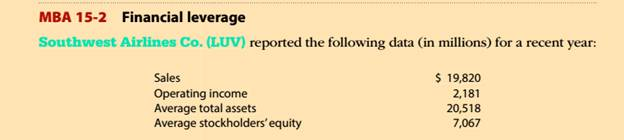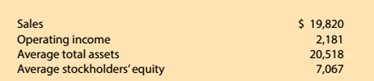Chapter 15, Problem 15.2.1MBA

Chapter
Section
Textbook ProblemFinancial leverage Southwest Airlines Co. (LUV) the following data (in millions) for a recent year:Compute the return on total assets.Round to one decimal place.

To determine

Concept Introduction:

Return on total Assets:

The Return on total assets is profitability ratio that measures the percentage of profit earned on average assets invested in the business. Return on asset is calculated by dividing the net income by average total assets. The formula to calculate Return on assets is as follows:

Return on assets = Net incomeAverage Total Assets

Note: Average total assets are calculated as an average of beginning and ending total assets. The formula to calculate the average total assets is as follows:

Average total Assets = (Beginning total assets + Ending total assets)2

To Calculate:

The return on total assets

Explanation

The return on total assets is calculated as follows:

 \$ in Millions Operating Income (A)

Still sussing out bartleby?

Check out a sample textbook solution.

See a sample solution

The Solution to Your Study Problems

Bartleby provides explanations to thousands of textbook problems written by our experts, many with advanced degrees!

Get Started

How is paid-in capital from sale of treasury stock usually shown on the balance sheet?

College Accounting, Chapters 1-27 (New in Accounting from Heintz and Parry)

Why was EDI devised?

Accounting Information Systems

NONCONSTANT GROWTH Milts Cosmetics Co.s stock price is 58.88, and it recently paid a 2.00 dividend. This divide...

Fundamentals of Financial Management, Concise Edition (with Thomson ONE - Business School Edition, 1 term (6 months) Printed Access Card) (MindTap Course List)